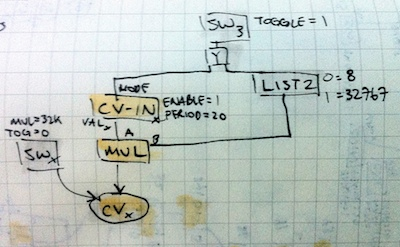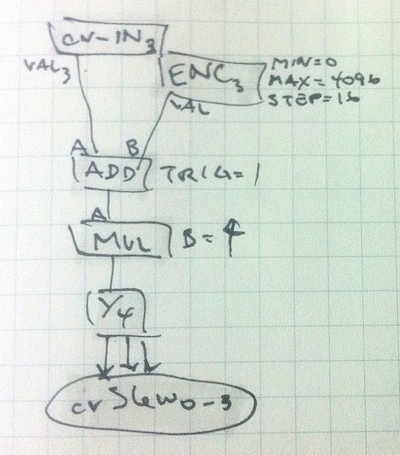Aleph Tutorial 7

Control Voltage

This Bees tutorial is designed to outline how to build scenes focussed around integration with hardware synthesizers, specifically through the use of control voltage. The two cv-input modes will be used to trigger events and control parameters in the aleph, while the cv-outs will send bees network data to a synthesizer.

First we’ll look at some simple cv utility modules, followed by a recreation of a section of the Buchla 266e Source of Uncertainty. This famed module generates a number of interesting random control voltages with varying methods of restricting that randomness. These will not be exact replicas, but should provide an interesting insight into how to construct more complex networks designed to interface with voltage controlled equipment.

CV Introduction

CV-IN

Aleph’s cv inputs are very similar to any other bees operator. Data at the inputs is available by the 010.CV-IN operator in two different modes, set by the 010.CV-IN/MODE input. When MODE = 0, the input responds to a continuous voltage in the range 0-10V. Anything outside this range is clamped at the limits – the corresponding output in bees ranges from 0 to 4095. When MODE = 1, the input expects a trigger/gate input of at least 2.5V which accomodates using most VCOs as a trigger source. The corresponding output in bees is a simple toggle from 0 to 1, and only sends a new value when the output changes. In order to function, the CV-IN operator needs to be set to ENABLE = 1 to begin ‘polling’ of the input jacks. The millisecond polling interval is set by the PERIOD input. A lower number (faster rate) will improve responsiveness of inputs though also increase CPU load – keep it above 20ms unless absolutely necessary.

cv_out

cv outs are not an operator in the bees network, but rather a set of parameters controlled by the currently loaded MODULE. In the waves and lines modules, each cv param has an associated cvSlew parameter to smoothly interpolate between values received from the bees network. By using or modulating the cvSlew parameters it’s possible to create smooth cv output from relatively few bees operators.

cvFuncGen

Trigger inputs to slewed envelopes.The CV-IN is set to trigger mode (MODE = 1) waiting for a high-state to trigger the cv outputs. The MUL operator is used to multiply up to full range (B = 32767), though if you prefer 5V envelopes set B = 16383. The first three switches manually trigger the functions high. Similar to the CV-IN a held press will maintain the function at it’s high state.

The right-most button is assigned to switch between CV-IN modes. Toggling to continuous mode changes the MUL scaling value (expecting 0-4095 input now) and the cv output will accurately follow the cv input.

The very simple connection of encoders to their respective cvSlew parameters allows the slew time for each function to be set independently. In continuous mode this changes the slew rate for the CV input.

cvQuantize

Cv quantizer with simultaneous outputs.

This scene uses (or abuses) the limitations of the aleph’s integer math to quantize incoming voltages to a set of musical values.Firstly the CV-IN is multiplied by 8 to be a full scale input (0-32767). A chromatic scale is found by using a combination of DIV and MUL both set to the integer size of a semitone. For CV this is ~273 (derived by dividing 32767 by 10 (octave range) then by 12 (semitones per octave) = 273). If using the internal aleph-waves synth you’ll want to use 256 instead, but we can compensate for this later.

The nature of the DIV and MUL combination is by dividing and discarding the remainder, as such rounding to the nearest integer, then multiplying back up to the previous level (minus the remainder).

In addition to semitones it’s interesting to have a value for which octave we’re in. To do so is the same as for semitones but dividing by the value of an octave (32767 / 10 = 3276). We round this number down to avoid errors later.

Next we can make a traditional major scale. Rather than dividing the input into 12 semitones per octave, we instead divide it into 7 tones. Firstly we discard any ‘octave’ information with a MOD which is the opposite of our octave approach – we’re discarding the main part of the division, and instead keeping the remainder. This is a value between 0 and 3276. This octave is then divided by 468 (3276 / 7(number of steps) = 468).

Now instead of simply multiplying this small number by 468 to reconstruct the scale, we need to apply our ‘scale’ otherwise, we’ll end up with a strange set of 7 notes equally far apart. To do so, make a LIST8 and fill it with the scale tones of the major scale: 0, 2, 4, 5, 7, 9, 11. Now when our 0-6 value from above is sent to the LIST8 it will choose the semitone equivalent value (in the range 0-12).

The final part of this puzzle is to add our semitone value (in the 0-3276 range) to the octave value from above. Make note of the input ordering on the ADD as the octave value needs to be received before the semitone.

Finally we can make a form of just intoned major scale. See the wikipedia page on just intonation for more information than i can possibly repeat.

Te process is quite simple as we’ll simply tap off the previous major scale output right after the LIST8 (giving a value 0-12) and instead of multiplying by 273 (a fixed distance semitone) we use a LIST16 containing values for just intoned semitones. For reference: 0, 219, 410, 655, 819, 1092, 1311, 1638, 1956, 2184, 2457, 2867.

Contrast this with the equal temperament scale of: 0, 273, 546, 819, 1092, 1365, 1638, 1911, 2184, 2457, 2730, 3003.

Add the octave as before, and assign the value to cv3.In order to test and trial the expected behaviour of this patch i created a random walk generator (METRO → RAND → ACCUM) to trigger the input. A more extensive example of this is described below in the 266fluctuating section. In order to interface the cv out values to the internal synth a little math is needed to translate from the 273/semitone of cv, to the 256/semitone of waves. Using a DIV B=53 → MUL B=50 gets close enough to sound ‘in tune’.

266fluctuating

“Fluctuating Random Voltages are continuously variable, with voltage control of bandwidth over the range of .05Hz to 50Hz, making possible changes that vary from barely perceptible movement to rapid fluctuation.”

To think about this more programatically we have a random number generator whose output is accumulated over time. In bees we’ll implement this with a METRO triggering a RANDOM at a fast speed. The ‘probable rate of change’ or ‘bandwidth’ control will set the bounds on the RANDOM’s output values. While this isn’t technically setting the ‘rate of change’ in our discrete system it gets us the same result.

We’ll make 3 copies of the fluctuator and assign the following interface:

• SW0-2: manual gates
• SW3: enable
• CV-IN0-2 & ENC0-2: probable rates of change
• CV-IN3 & ENC3: cvSlew rate
• cvouts0-2: fluctuating outputs

The following is a block diagram of the signal flow of one section:The first section is the repeated part of each generator. The METRO is enabled by SW3 in toggle mode. This repeating clock is our substitute for a continuous signal, so it’s run at a high rate of 50Hz (PERIOD = 20). The clock is sent to all three sections and then GATEd again using the aleph’s switches in toggle mode.

When the GATE is open the RANDOM operator is triggered generating a number which is added to the ACCUM operator, and finally sent to the cv parameter. The range of the RANDOM generator is controlled by the corresponding encoder (0,1,2) and CV-IN (0,1,2) for each section. The encoder and cv are added together, then split to the RANDOM/MAX input, and inverted (with a MUL, B = -1) to the RANDOM/MIN input. This has the effect of creating a bipolar range where the ACCUM can be either increased or decreased by the RANDOM op.

The maximum change per METRO tick is the maximum CV-IN (4095) plus the maximum ENC (4096) which gives 8191 – roughly equivalent to 2.5V per tick.The second element of the scene is a simple setup showing encoder and voltage-control of the cvSlew params. It should be rather self-explanatory with a simple ADD triggering on new CV-IN or ENC settings, then being multiplied up to full scale (0-32767) and distributed to all three cvSlew params with a SPLIT4.

A completed 3 section version is included in the aleph-bees distribution.

Going further

• cvFuncGen: link CV-IN0 to all cv outs with varying rates
• cvQuantize: change the scale
• 266: add sections 2 and 3
• 266: link cvSlew to the rate of change
• 266: lower the METRO speed for quantized stepping

Tutorial Reference

Tutorial 0: Getting Started with Bees

Tutorial 1: Making a Network

Tutorial 2: A Simple Synthesizer

Tutorial 3: Connecting a Controller

Tutorial 4: Sequencing & Modulation

Tutorial 5: Presets, Presets, Presets!

Tutorial 6: More Presets

Tutorial 7: Control Voltage